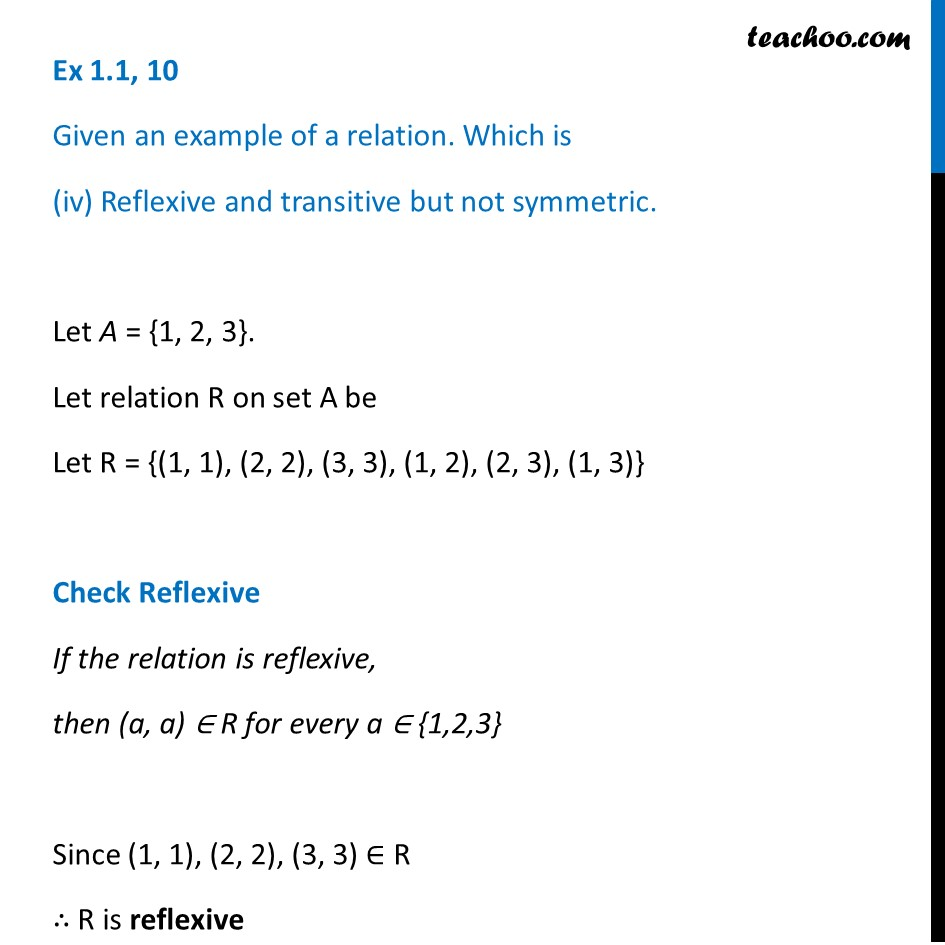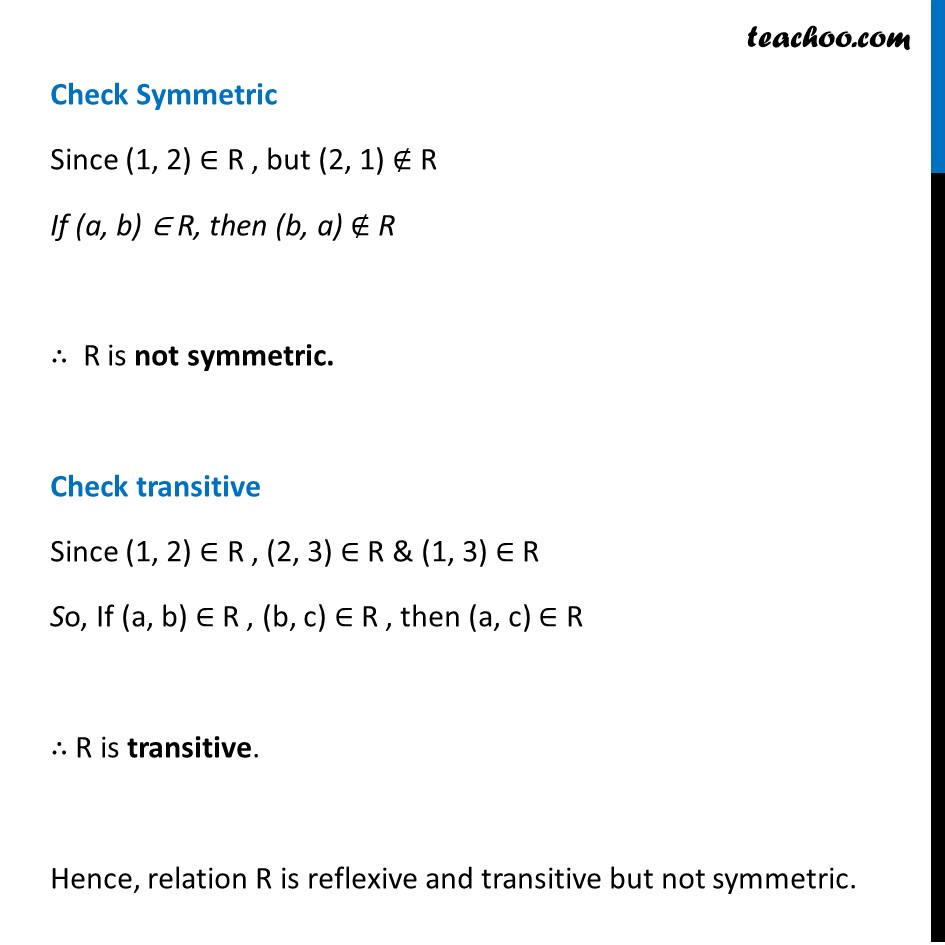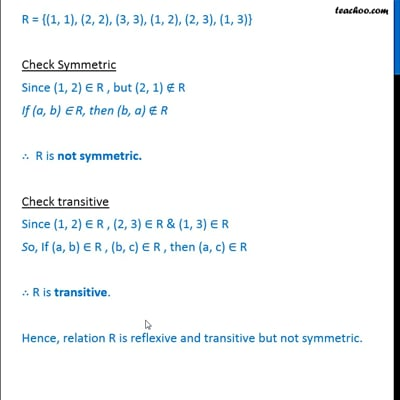Ex 1.1

Chapter 1 Class 12 Relation and Functions
Serial order wiseThis video is only available for Teachoo black users

Learn in your speed, with individual attention - Teachoo Maths 1-on-1 Class

### Transcript

Ex 1.1, 10 Given an example of a relation. Which is (iv) Reflexive and transitive but not symmetric. Let A = {1, 2, 3}. Let relation R on set A be Let R = {(1, 1), (2, 2), (3, 3), (1, 2), (2, 3), (1, 3)} Check Reflexive If the relation is reflexive, then (a, a) ∈ R for every a ∈ {1,2,3} Since (1, 1), (2, 2), (3, 3) ∈ R ∴ R is reflexive Check Symmetric Since (1, 2) ∈ R , but (2, 1) ∉ R If (a, b) ∈ R, then (b, a) ∉ R ∴ R is not symmetric. Check transitive Since (1, 2) ∈ R , (2, 3) ∈ R & (1, 3) ∈ R So, If (a, b) ∈ R , (b, c) ∈ R , then (a, c) ∈ R ∴ R is transitive. Hence, relation R is reflexive and transitive but not symmetric.